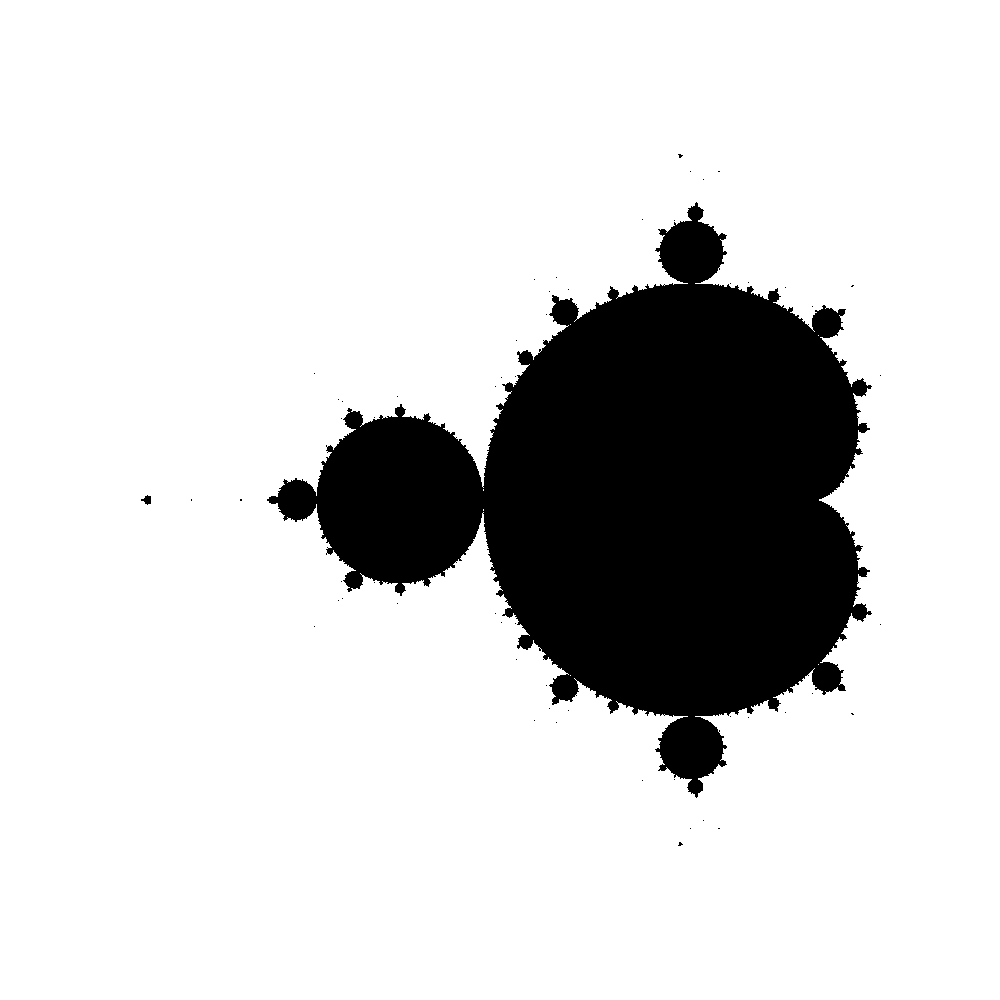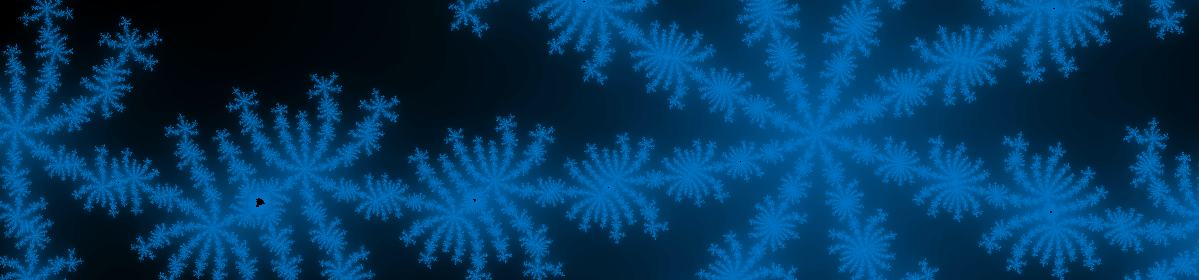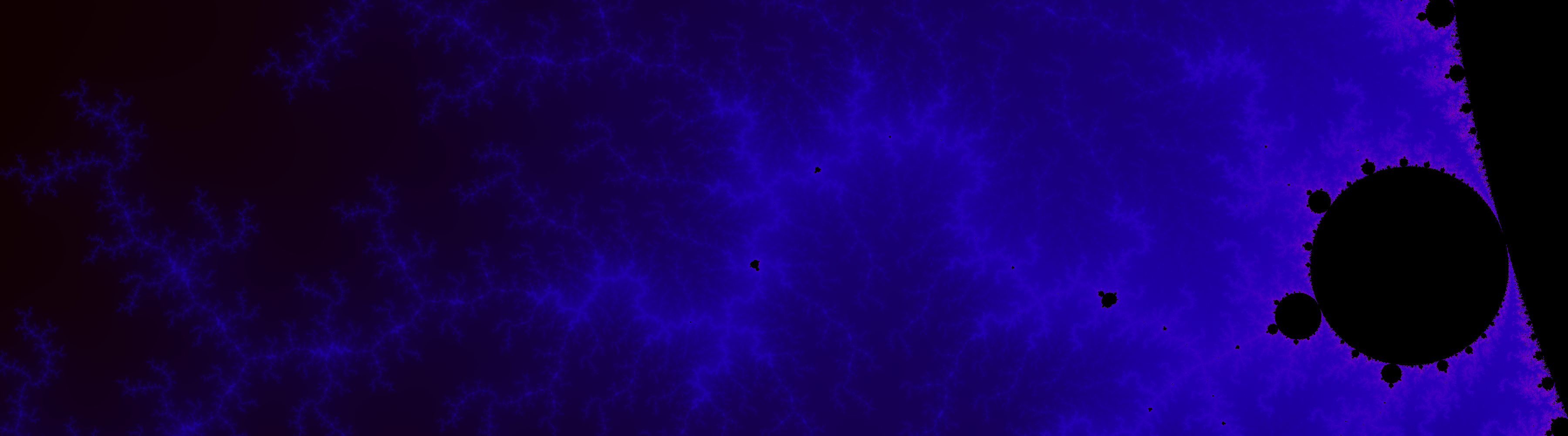# Mandelbrot

The Mandelbrot set is the set of complex numbers, $$c$$, such that the series
$$z_{n+1} = z_n^2 + c$$
does not diverge as $$n\rightarrow \infty$$. These values of $$c$$ can then be plotted in the complex plane to produce some interesting images. As each pixel in the image is an independent calculation this is a good problem for getting familiar with parallel computing. The following images were generated with the code at https://github.com/smsolivier/Mandelbrot.